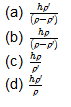# JEE and NEET Multiple Choice questions (Mixed)

PART A

Question 1
A ball is thrown vertically upwards with a velocity u from top of a tower. It strikes the ground with a velocity 3u. The time taken by the ball to reach the ground is given by
(a) u/g
(b) 2u/g
(c) 3u/g
(d) 4u/g

Question 2
A body slides down an inclined plane of inclination . The coefficient of friction down the plane varies in the direct proportion to the distance moved down the plane(=kx). The body will move down the plane with
(a) constant acceleration =gsin
(b) constant acceleration =(gsin-gcos)
(c) constant retardation =(gcos-gsin)
(d) variable acceleration that first decreases from gsin to zero and that becomes negative.

Question 3
A escalator is moving downwards with a uniform speed u. A man of mass m is running upwards on it at a uniform speed v. If the height of escalator is h, the work done by man in going up the escalator is
(a) zero
(b) mgh
(c) mghu/(v-u)
(d) mghv/(v-u)

Question 4
A particle moves in a circular orbit with a uniform angular speed. However , the plane of the circular orbit is itself rotating at a constant angular speed. We may then say that
(a) the angular velocity as well as angular acceleration of particle are both constant.
(b) neither the angular velocity nor the angular acceleration of the particle are constant.
(c) the angular velocity of the particle varies but its angular acceleration is constant.
(d) the angular velocity of the particle remains constant but the angular acceleration varies.
Question 5
Two objects of mass m and 4m are at rest at infinite separation. They move towards each other under mutual gravitational attraction. Then at separation r, which one of the following is true.
(a) the total energy of the system is not zero
(b) the force between them is not zero
(c) the center of mass of the system is at rest
(d) all the above are true

PART B

Question 1

A body of density enters a tank of water of density ' after falling through height h. The maximum depth to which it sinks in water isQuestion 2.
A charged particle q is shot towards another charged particle Q which is fixed, with speed v. It approaches Q up to a closest distance r and then returns. If q were given a speed 2v , the distance of approach would be
(a) r
(b) 2r
(c) r/2
(d) r/4

Question 3.
The Young’s modulus of a perfectly rigid body is
(a)  zero
(b)  unity
(c)  infinity
(d)  may have any finite non zero value

Question 4.
When an EM wave enters an ionised layer of earth atmosphere present in ionosphere
(a)  the electron cloud will not oscillate in the electric field of the wave
(b) the electron cloud will oscillate in the electric field of wave in  phase of sinusoidal EM wave
(c) the electron cloud will oscillate in the electric field of wave in the opposite phase of sinusoidal EM wave
(d) the electron cloud will oscillate in the electric field of wave with a phase retardation of 90 degree for a sinusoidal EM wave

Question 5.
Two bodies are charged by rubbing one against the other. During the process one becomes positively charged while other becomes negatively charged. then
(a) mass of each body remains unchanged
(b) mass of each body changes marginally
(c) mass of each body changes slightly and hence the total mass
(d) mass of each body changes slightly but total mass remains the same

PART A

1. b
2. d
3. d
4. c
5. d

PART B
1. (a)
2. (d)
3. (c)
4. (d)